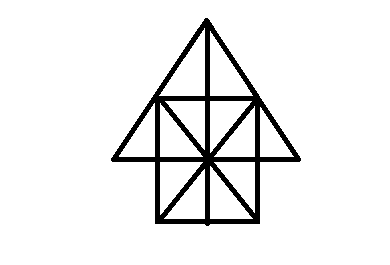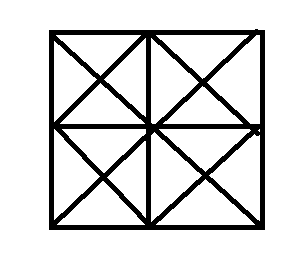• 1
20
Correct
Incorrect
• 2
24
Correct
Incorrect
• 3
28
Correct
Incorrect
• 4
32
Correct
Incorrect
• Workspace
• Discuss

• 1
16
Correct
Incorrect
• 2
17
Correct
Incorrect
• 3
26
Correct
Incorrect
• 4
30
Correct
Incorrect
• Workspace
• Discuss

• 1
16
Correct
Incorrect
• 2
14
Correct
Incorrect
• 3
8
Correct
Incorrect
• 4
12
Correct
Incorrect
• Workspace
• Discuss

## Q: In question below shown, count the number of triangle and squares.21 05d2c46522bb9b81db4a97ee6

• 1
26 triangles, 5 squares
Correct
Incorrect
• 2
28 triangles, 5 squares
Correct
Incorrect
• 3
26 triangles,6 squares
Correct
Incorrect
• 4
28 triangles, 6 squares
Correct
Incorrect
• Workspace
• Discuss

• 1
27
Correct
Incorrect
• 2
25
Correct
Incorrect
• 3
23
Correct
Incorrect
• 4
21
Correct
Incorrect
• Workspace
• Discuss

• 1
12
Correct
Incorrect
• 2
18
Correct
Incorrect
• 3
22
Correct
Incorrect
• 4
26
Correct
Incorrect
• Workspace
• Discuss

• 1
21
Correct
Incorrect
• 2
23
Correct
Incorrect
• 3
25
Correct
Incorrect
• 4
27
Correct
Incorrect
• Workspace
• Discuss

## Q: In the following questions, count the number of triangles and squares in the given figure.3 05d2c452b2bb9b81db4a97ea2

• 1
44 triangles,10 squares
Correct
Incorrect
• 2
14 triangles, 16 squares
Correct
Incorrect
• 3
27 triangles,6 squares
Correct
Incorrect
• 4
36 triangles, 9 squares
Correct
Incorrect
• Workspace
• Discuss

## Answer : 1. "44 triangles,10 squares"Explanation :

Error Reported Successfully

Error Reported Successfully

Error Reported Successfully

Error Reported Successfully

Error Reported Successfully